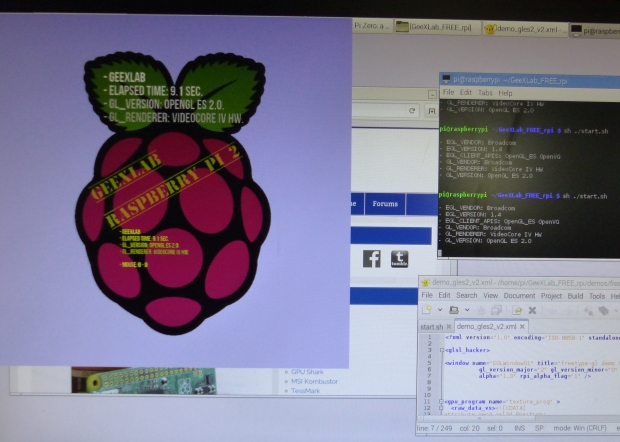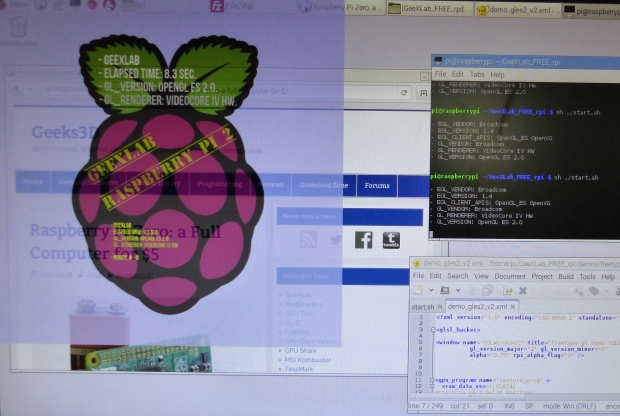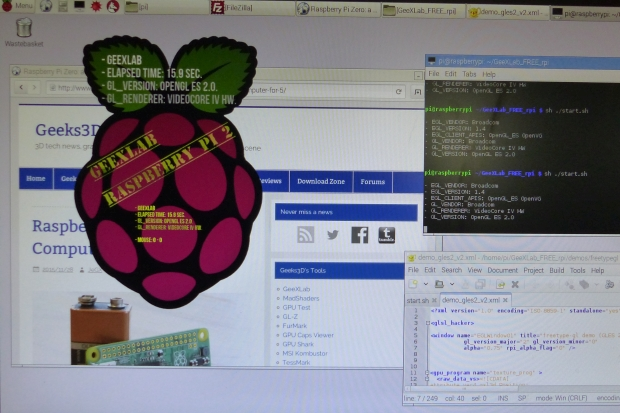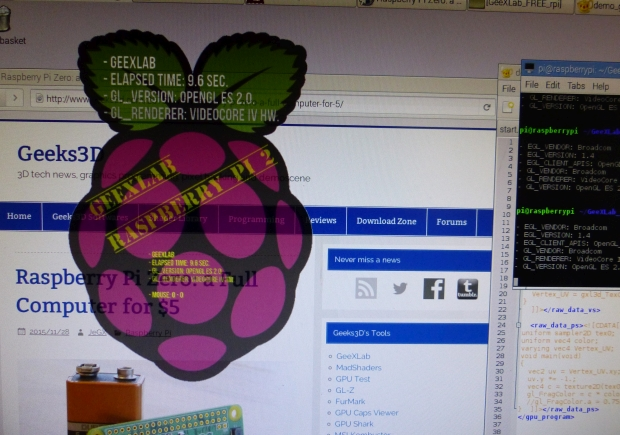# How to Control the Window Opacity on the Raspberry PiOn the Raspberry Pi there is a very nice feature that allows to create very cool shaped windows: the control of the opacity of the 3D window.

The control of the opacity is currently done in GeeXLab in the window XML node with two attributes:

• alpha
• rpi_alpha_flag

The default values are alpha=1.0 and rpi_alpha_flag=1 :

```<window name="EGLWindow01" title="freetype-gl demo (GLES 2.0)"
width="800" height="800"
gl_version_major="2" gl_version_minor="0"
alpha="1.0" rpi_alpha_flag="1" />
```

Let’s take a simple demo that displays the Raspberry Pi logo (a PNG image with transparency) and let’s see how rpi_alpha_flag acts on the opacity.

The demo is available in the code sample pack (gles2/freetypegl/demo_gles2_v2.xml). The code sample pack as well as GeeXLab for the Raspberry Pi can be downloaded from THIS PAGE.

### rpi_alpha_flag=1

rpi_alpha_flag=1 and alpha=1.0 is the default mode: the window is fully opaque.With alpha less than 1.0, the whole window is transparent.### rpi_alpha_flag=0

When rpi_alpha_flag=0, the alpha value has no longer impact. In this mode, the alpha value outputed by the pixel shader on the default framebuffer is used to control the transparency.

On the following screenshot, the Raspberry Pi PNG image logo is fully transparent outside the raspberry and fully opaque inside. The pixel shader outputs the following alpha:

```vec4 raspberry_tex = texture2D(tex0, uv);
gl_FragColor.rgb = raspberry_tex.rgb;
gl_FragColor.a = raspberry_tex.a;
```### rpi_alpha_flag=2

rpi_alpha_flag=2 can be seen as a mix between the previous modes. If alpha=1.0, we get:If alpha is less than 1.0, we get a transparent window without touching the alpha value of the pixel shader: